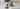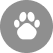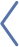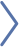# Cat Calorie Calculator: How to Calculate Your Cat’s Daily Calorie IntakeJust like with humans, a cat’s calorie requirements depend on several factors. Calories, the technical term for energy from food, are required for essential processes such as digestion, respiration, nervous and circulatory system control, and other normal bodily functions. In addition to the body’s basic needs, a cat’s daily intake is determined by age, sex, activity level, and body condition.

For example:

• Young cats, those who have not been spayed or neutered, active cats, sick cats, and underweight cats need more calories.
• Older cats, spayed or neutered cats, inactive cats, and overweight or immobile cats need fewer calories.

## How to Calculate How Much Your Cat Can Eat Per Day

Feeding too little food can deprive your cat of necessary energy and feeding too much can result in obesity. To determine how much food your cat should eat per day, follow these steps.

You can weigh your cat at your veterinarian’s office or at home. The most accurate way to weigh your cat at home is to weigh yourself while holding your cat, then weigh yourself alone and subtract the second total from the first total. For example, if you and your cat weight 161 pounds and you alone weigh 150 pounds, then your cat weighs 11 pounds.

### 2. Use a cat calorie calculator to calculate resting energy requirements (RER). (Table 1):

 Body weight (lbs) Body weight (kg) RER ( calories per day) 1 0.5 39 2 0.9 65 3 1.4 88 4 1.8 110 5 2.3 130 6 2.7 149 7 3.2 167 8 3.6 184 9 4.1 200 10 4.5 218 11 5 234 12 5.5 250 13 5.9 265 14 6.4 280 15 6.8 295 16 7.3 310 17 7.7 324 18 8.2 339 19 8.6 353 20 9.1 366 25 11.4 433

Table 1. The cat calorie calculator that many veterinarians use to calculate RER calorie requirements is provided by Hill’s Pet Nutrition. NOTE: The “calorie” we refer to is actually “kilocalorie.”

### 3. Choose a factor (Table 2) based on your cat’s energy needs and multiply it by the RER.

The RER fulfills the cat’s basic needs, but does not account for things like activity level or other factors. To do so, the RER number is multiplied by factors to estimate the cat’s total daily energy needs. Some cats need less than the RER and some need nearly double the RER. Choose the factor below that fits your cat.

 Factors to estimate feline daily energy needs: Neutered adult cat = 1.2 x RER Intact adult cat = 1.4 x RER Inactive/obese prone cat = 1 x RER Weight loss for cat = 0.8 x RER for ideal weight Weight gain for cat = 1.8 x RER for ideal weight Kitten 0 to 4 months = 2.5 x RER Kitten 4 months to 1 year = 2 x RER

Table 2. Factors used to calculate calorie needs in cats.

### 4. Determine how many calories are in your cat’s food and treats.

You can find this information on the food package or online. In general, dry food has more calories per ounce than canned food. You may see the calorie listing as “calorie” or as “kilocalorie,” which is the same thing. Once you know the calories in what you are feeding, you can figure out how much your cat needs. Treats should account for less than 10% of your cat’s calorie intake.

Every food has a unique calorie count. Take human food, for example: 1 cup of cooked green beans has about 44 calories, but a cup of macaroni salad is around 360 calories. Cat food is the same way in that some formulas are more calorie dense than others (so you need to feed less).

### 5. Figure out the amounts to feed.

If you feed your cat two meals a day, subtract the calorie count of any treats and divide the remainder by 2 to determine how many calories your cat should get for each meal. Use the calorie count for your cat’s specific food to measure properly.

## Examples of How to Use the Cat Calorie Calculator

Here are a couple examples of how to use the cat calorie calculator:

### Example 1

Let’s pretend you have a 9-pound adult cat. First, look at Table 1 for the amount of basic calories your cat can eat then look for the factor in Table 2 to determine real needs. When you look at Table 1, you can see that they can eat 200 calories per day. Your cat is a normal indoor and not particularly active cat, and you look at table 2 and see that the factor for calculation of their daily energy needs is “1,” so you multiply 200 x 1, which is 200 calories. You don’t feed treats and only feed a dry food that is 200 calories per cup. So… your cat can eat 1 cup of food per day.

### Example 2

You have a 12-pound, very active, intact male cat. When you look at Table 1, you can see that he can eat 250 calories per day. Because he is intact and active, you see in Table 2 that the factor to use to calculate calorie requirements is 1.4 x RER. So, you take 1.4 and multiply by 250 = 350 calories per day. So, this cat can eat 350 calories per day.

### Example 3

You are calculating calorie intake for a 4-pound, 7-month-old, very active female kitten. When you look at the cat calorie calculator in Table 1, you can see that she can eat 110 calories per day. Because she is a kitten (4 months to a year old), you see in Table 2 that the factor you use to calculate calorie requirements is 2 x RER. So, you take factor two and multiply by 110 = 220 calories. This kitten can eat 220 calories per day.

## How to Calculate Calories for Weight Loss

If your cat is overweight, it is healthier to help them reach an ideal weight.

Here is an example of how to calculate calories for weight loss. During a recent visit to the vet, they point out that your cat’s body condition is considered too high. Their belly is sagging and when you view it from above, there is no “hour-glass” to their figure. The cat’s weight was 19 pounds. When we look at Table 1, the basic RER requirements for a 19-pound cat is 353 calories. When you look at Table 2, you want “weight loss” which gives us a factor = 0.8 x RER to attain an ideal weight.

The calculation is 353 calories x 0.8 = 282 calories. For this 19-pound cat, 282 calories is the appropriate amount for losing weight.

## How to Calculate Calories for Weight Gain

Some cats can be too thin and may need to put on some extra pounds. Thin cats have a body condition reflected in a narrowed waist, protruding rib cage, and exposed bony processes of the spine.

Here is an example of how to calculate calories for weight gain. Let’s say you have a 6-pound cat and you want to calculate the energy requirements for weight gain. When we look at Table 1, the basic RER requirements for a 6-pound cat is 149 calories. When you look at Table 2, you see a factor of 1.8 x RER for ideal weight.

The calculation is 149 calories x 1.8 = 268.2 calories. This 6-pound cat needs to eat approximately 268 calories to gain weight.

## Rule-of-Thumb Cat Calorie Calculator

A rule-of-thumb for calorie requirements for a normal adult cat is 20 to 33 calories per pound depending on their energy level. A 10-pound indoor cat should eat about 200 calories and an outdoor active cat can eat about 330 calories per day.0 paws up